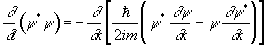# 1.[5pt]Conservation of probability

a)                  Derive the equationwhere y is any solution to the Schrödinger equation.

b)                  Show explicitely that for stationary states each side of the above equation is identically zero.